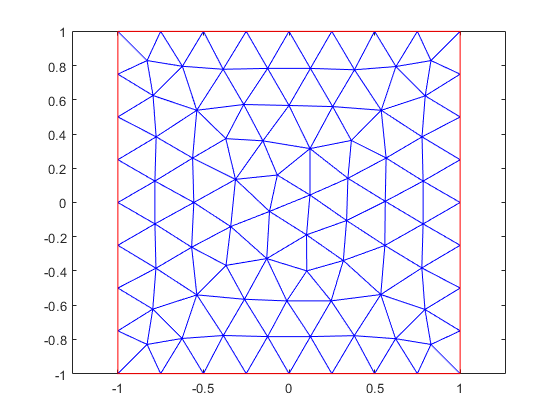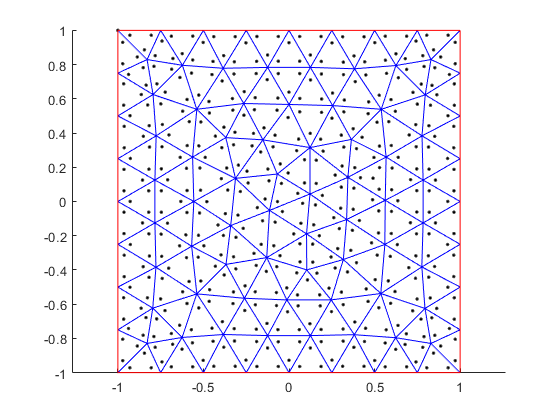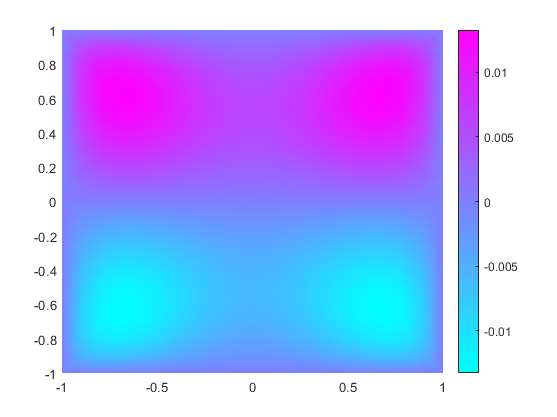## Specify Nonconstant PDE Coefficients

When solving PDEs with nonconstant coefficients, specify these coefficients by using function handles. This example shows how to write functions to represent nonconstant coefficients for PDE problems.

### Geometry and Mesh

Create a model.

`model = createpde;`

Include a unit square geometry in the model.

`geometryFromEdges(model,@squareg);`

Generate a mesh with a maximum edge length of 0.25. Plot the mesh.

```generateMesh(model,'Hmax',0.25); pdemesh(model)```### Function for Nonconstant Coefficient f

Write a function that returns the value $f\left(x,y\right)={x}^{2}\mathrm{sin}\left(y\right)$ for the nonconstant coefficient f. The function must accept two input arguments, `location` and `state`. The solvers automatically compute and populate the data in the `location` and `state` structure arrays and pass this data to your function. To visualize the `location` data used by the toolbox, add the scatter plot command to your function.

```function fcoeff = fcoefffunc(location,state) fcoeff = location.x.^2.*sin(location.y); scatter(location.x,location.y,'.','black'); hold on end ```Specify the PDE coefficients using the function that you wrote for the f coefficient.

```specifyCoefficients(model,'m',0, ... 'd',0, ... 'c',1, ... 'a',0, ... 'f',@fcoefffunc);```

Apply the Dirichlet boundary condition u = 0 for all edges of the square.

`applyBoundaryCondition(model,'dirichlet','Edge',1:4,'u',0);`

Solve the equation and plot the solution.

```results = solvepde(model); figure pdeplot(model,'XYData',results.NodalSolution)```### Anonymous Function for a PDE Coefficient

If the dependency of a coefficient on coordinates, time, or solution is simple, you can use an anonymous function to represent the nonconstant PDE coefficient. Thus, you can implement the dependency shown earlier in this example as the `fcoefffunc` function, as this anonymous function.

`f = @(location,state)location.x.^2.*sin(location.y);`

Specify the PDE coefficients.

```specifyCoefficients(model,'m',0, ... 'd',0, ... 'c',1, ... 'a',0, ... 'f',f);```

If a function that represents a nonconstant PDE coefficient requires more arguments than `location` and `state`, follow these steps:

1. Write a function that takes the `location` and `state` arguments and the additional arguments.

2. Wrap that function with an anonymous function that takes only the `location` and `state` arguments.

For example, define the coefficient f as $f\left(x,y\right)=a{x}^{2}\mathrm{sin}\left(by\right)+c$. First, write the function that takes the arguments `a`, `b`, and `c` in addition to the `location` and `state` arguments.

```function fcoeff = fcoefffunc_abc(location,state,a,b,c) fcoeff = a*location.x.^2.*sin(b*location.y) + c; end ```

Because functions defining nonconstant coefficients must have exactly two arguments, wrap the `fcoefffunc_abc` function with an anonymous function.

```fcoefffunc_add_args = ... @(location,state) fcoefffunc_abc(location,state,1,2,3);```

Now you can use `fcoefffunc_add_args` to specify the coefficient f.

```specifyCoefficients(model,'m',0, ... 'd',0, ... 'c',1, ... 'a',0, ... 'f',fcoefffunc_add_args);```